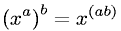Equations > Algebra > Exponents/Powers > Power Rule of Exponents

### Power Rule of ExponentsLatex Code:

MathML Code:

 ${\left({x}^{a}\right)}^{b}={x}^{\left(\mathrm{ab}\right)}$

MathType 5.0: